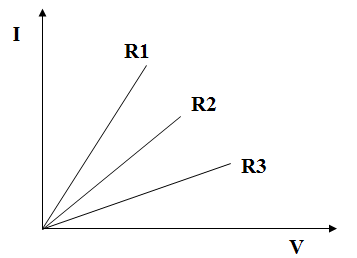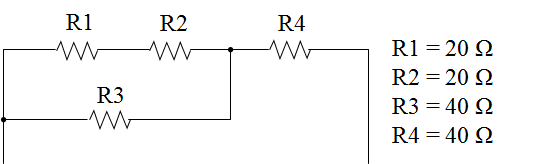### Exercises Basics Electrics

Part 1: Multiple Choice - only one statement is correct

At a power resistor with 10 Ω a power of 5 W is converted. What power is converted when the applied voltage is doubled?

Which statement agrees with the resistance curves shown below?Resistors curves

Resistors of different sizes are connected in parallel. Which statement is correct?

How does the power behave when the resistance is halved?

Which statement about the circuit below is correct?Resistors – Mixed connections